## Second Derivative Test

Supposeis a Function ofwhich is twice Differentiable at a Stationary Point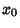.

1. If, thenhas a Relative Minimum at.

2. If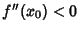, thenhas a Relative Maximum at.

The Extremum Test gives slightly more general conditions under which a function with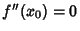is a maximum or minimum.

Ifis a 2-D Function which has a Relative Extremum at a pointand has Continuous Partial Derivatives at this point, thenand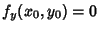. The second Partial Derivatives test classifies the point as a Maximum or Minimum. Define the Discriminant as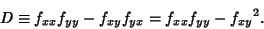1. If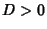,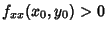and, the point is a Relative Minimum.

2. If,, and, the point is a Relative Maximum.

3. If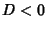, the point is a Saddle Point.

4. If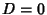, higher order tests must be used.

See also Discriminant (Second Derivative Test), Extremum, Extremum Test, First Derivative Test, Global Maximum, Global Minimum, Hessian Determinant, Maximum, Minimum, Relative Maximum, Relative Minimum, Saddle Point (Function)

References

Abramowitz, M. and Stegun, C. A. (Eds.). Handbook of Mathematical Functions with Formulas, Graphs, and Mathematical Tables, 9th printing. New York: Dover, p. 14, 1972.